Note for chapter 1 Data Visualization (数据可视化)

Vacabulary for this chapter
left skewed 左倾斜
symmetric 对称
right skewed 右倾斜
outliers 异常值，极端值

## Qualitative Variables and Quantitative Variables

Categorical (qualitative) variables 属性变量: individuals are placed into groups or categories

Quantitative variables 定量变量: numerical values where arithmetic operations such as adding and averaging make sense.

## Contingency Table (列联表)

Sometimes, contingency tables are used to display the frequency distribution of multiple variables.

Example: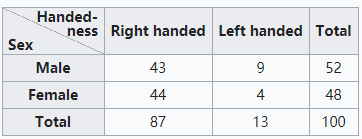## Bar Chart (条形图)

Bar charts are preferred as:

• It is easier to draw (especially when you need to do it by hand)
• It is easier to compare the size of various categories (especially when the difference is not that significant)
• It can be used if your categories do not add up to “a whole”
• You can label the name and value of each categories (especially if you have many) without making the graph looking too messy.

Example: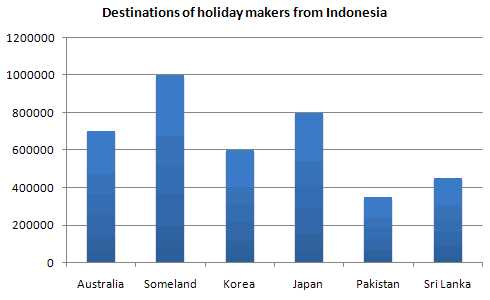## Pie Chart (饼图)

Pie chart is preferred when:

• Making comparison for 2~3 different data points with very different amounts of information
• Show relationship of parts out of a whole.

Example: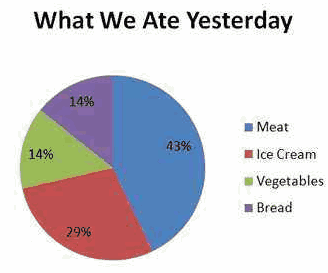## Line Graph (线图)

Line graph is useful to display the change of values over time.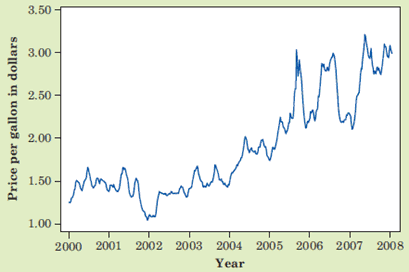## Histogram (直方图)

Histogram shows the frequency distribution of values, looks similar as bar chart.

• The horizontal axis is the numerical number line
• The non-overlapping classes of same width are represented by rectangles
• Heights (hence the total area) of each rectangle represents the frequency/counts in each particular class.
• The overall distribution of data is nicely shown if properly drawn.

Example: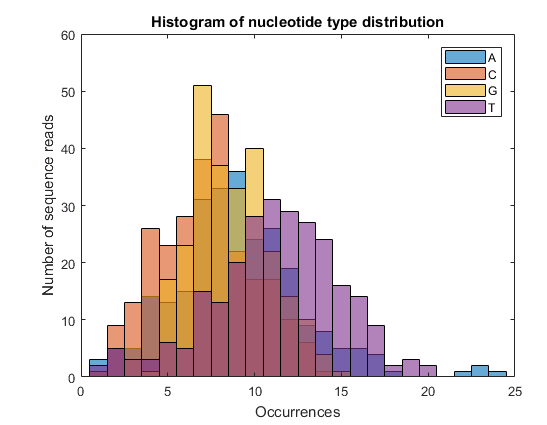Bar Chart 和 Histogram 的区别：
Bar Chart 的横轴是属性变量（qualitative variables），Histogram 的横轴是数字区间（Quantitative variables）

• Left Skewed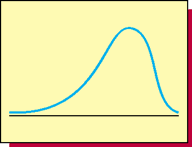• Symmetric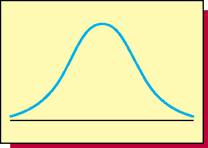• Right Skewed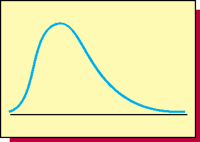## Scatter plot (散点图)

Scatter plot helps to reveal the relationship between two variables.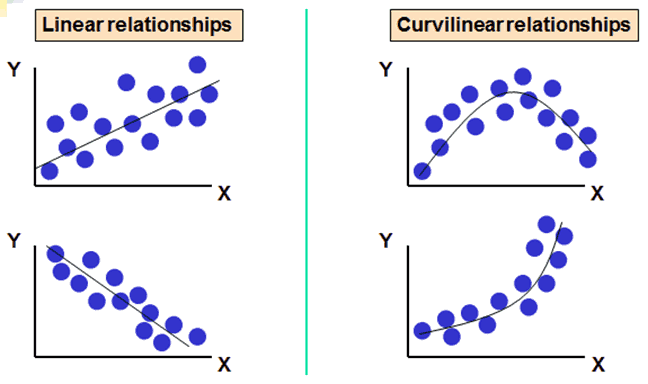## Boxplot (盒形图)

Boxplot displays the position of the minimum, maximum and outlier values, as well as the first, second and third quartiles.

Boxplots can be easily put side-by-side for comparison.

For a set of measurements arranged in increasing order, the $\mathbf{p}^{t h}$ percentile(百分位点) is a value such that p percent of the measurements fall at or below the value and (100-p) percent of the measurements fall at or above the value

• The first quartile $Q_{1}$ is the $\mathbf{25}^{th}$ percentile
• The second quartile (or median) $M_{d}$ is the $\mathbf{50}^{th}$ percentile
• The third quartile $Q_{3}$ is the $\mathbf{75}^{th}$ percentile
• The interquartile range (IQR 四分位距) is $Q_{3}$ - $Q_{1}$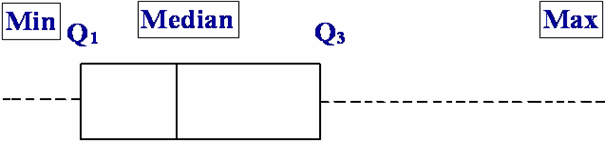The box plots the:

• first quartile, $Q_{1}$;
• median, $M_{d}$;
• third quartile, $Q_{1}$

Limits, located 1.5 x IQR away from the quartiles:

• lower limit= $Q_{1}$ – (1.5 x IQR)
• upper limit= $Q_{3}$ + (1.5 x IQR)

The whiskers: 2 dashed lines

• A dashed line drawn from the box below Q1 down to the smallest measurement between the lower and upper limits
• Another dashed line drawn from the box above Q3 up to the largest measurement between the lower and upper limits

Outliers lie beyond the limits of the box-and-whiskers plot. Outliers are measurements that are very different from most of the other measurements. Plot each outlier using the symbol *.

Example: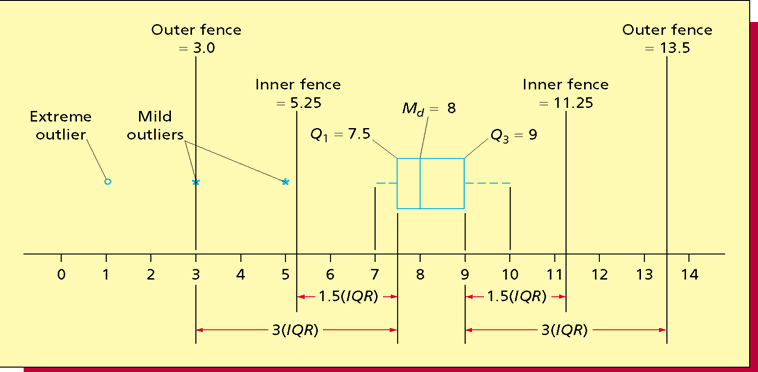The box plots the:

• first quartile, $Q_{1}$;
• median, $M_{d}$;
• third quartile, $Q_{1}$
• inner fences, located 1.5IQR away from the quartiles:
• = $Q_{1}$ – (1.5 x IQR)
• = $Q_{3}$ + (1.5 x IQR)
• outer fences, located 3IQR away from the quartiles:
• = $Q_{1}$ – (3 x IQR)
• = $Q_{3}$ + (3 x IQR)
• The “whiskers” are dashed lines that plot the range of the data
• A dashed line drawn from the box below $Q_{1}$ down to the smallest measurement
• Another dashed line drawn from the box above $Q_{3}$ up to the largest measurement
• Note: $Q_{1}$, $M_{d}$, $Q_{3}$, the smallest value, and the largest value are sometimes referred to as the five number summary

Outliers lie beyond the fences of the box-and-whiskers plot

• Measurements between the inner and outer fences are mild outliers (轻微异常)
• Measurements beyond the outer fences are severe outliers (严重异常)